Share

# RS Aggarwal solutions for Secondary School Class 10 Maths chapter 12 - Circles [Latest edition]

Course
Textbook page

#### Chapters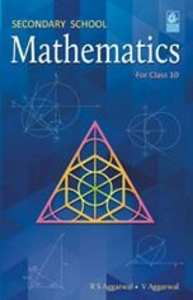## Chapter 12: Circles

#### RS Aggarwal solutions for Secondary School Class 10 Maths Chapter 12 Circles

Q 1

Find the length of tangent drawn to a circle with radius 8 cm form a point 17 cm away from the center of the circle

Q 2

A point P is 25 cm away from the center of a circle and the length of tangent drawn from P to the circle is 24 cm. Find the radius of the circle.

Q 3

Two concentric circles are of radii 6.5 cm and 2.5 cm. Find the length of the chord of the larger circle which touches the smaller circle.

Q 4

In the given figure, a circle inscribed in a triangle ABC, touches the sides AB, BC and AC at points D, E and F Respectively. If AB= 12cm, BC=8cm and AC = 10cm, find the length of AD, BE and CF.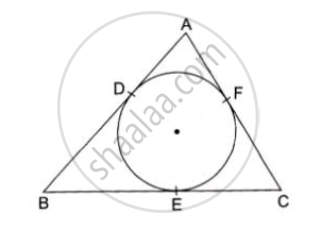Q 5

In the given figure, a circle touches all the four sides of a quadrilateral ABCD whose three sides are AB = 6cm, BC=7cm and CD=4 cm. Find AD.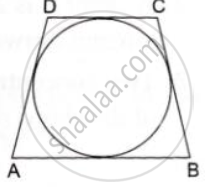Q 6

In the given figure, the chord AB of the larger of the two concentric circles, with center O, touches the smaller circle at C. Prove that AC = CB.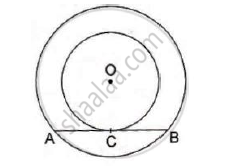Q 7

From an external point P, tangents PA and PB are drawn to a circle with center O. If CD is the tangent to the circle at a point E and PA = 14cm, find the perimeter of ΔPCD.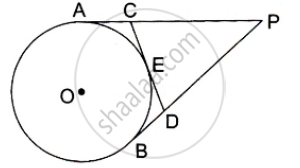Q 8

A circle is inscribed in a ΔABC touching AB, BC and AC at P, Q and R respectively. If AB = 10 cm, AR=7cm and CR=5cm, find the length of BC.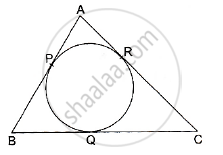Q 9

In the given figure, PA and PB are the tangent segemtns to a circle with centre O. Show that he points A, O, B and P are concyclic.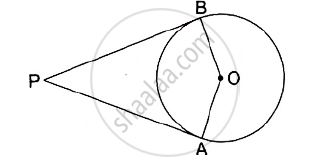Q 10

In the given figure, an isosceles triangle ABC, with AB = AC, circumscribes a circle. Prove that point of contact P bisects the base BC.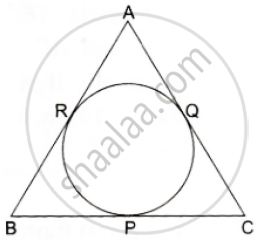Q 11

In the given figure, O is the centre of the two concentric circles of radii 4 cm and 6cm respectively. AP and PB are tangents to the outer and inner circle respectively. If PA = 10cm, find the length of PB up to one place of the decimal.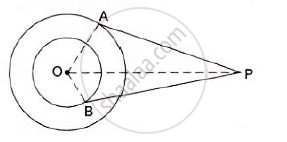Q 12

In the given figure, a triangle ABC is drawn to circumscribe a circle of radius 3 cm such that the segments BC and DC into which BC is divided by the point of contact D, are of
lengths 6cm and 9cm respectively. If the area of 2 ΔABC = 54cm2 then find the lengths of sides AB and AC.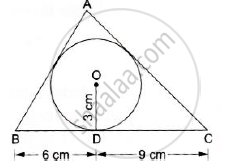Q 13

PQ is a chord of length 4.8 cm of a circle of radius 3cm. The tangents at P and Q intersect at a point T as shown in the figure. Find the length of TP.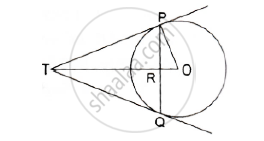Q 14

Prove that the line segment joining the points of contact of two parallel tangents of a circle, passes through its centre.

Q 15

In the given figure, a circle with center O, is inscribed in a quadrilateral ABCD such that it touches the side BC, AB, AD and CD at points P, Q, R and S respectively. If AB = 29cm, AD = 23cm, ∠B = 90° and DS=5cm then find the radius of the circle.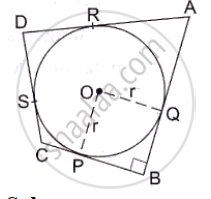Q 16

In the given figure, O is the centre of the circle and TP is the tangent to the circle from an external point T. If ∠PBT = 30° , prove that BA : AT = 2 : 1.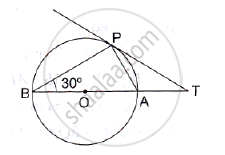#### RS Aggarwal solutions for Secondary School Class 10 Maths Chapter 12 Circles

Q 1

In the adjoining figure, a circle touches all the four sides of a quadrilateral ABCD whose sides are AB=6cm, BC=9cm and CD=8 cm. Find the length of side AD.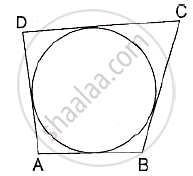Q 2

In the given figure, PA and PB are two tangents to the circle with centre O. If ∠APB = 50° then what is the measure of ∠OAB.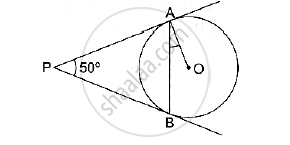Q 3

In the given figure, O is the centre of a circle. PT and PQ are tangents to the circle from an external point P. If ∠TPQ = 70° , find the ∠TRQ.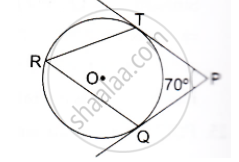Q 4

In the given figure common tangents AB and CD to the two circles with centres O1 and O2 intersect at E. Prove that AB=CD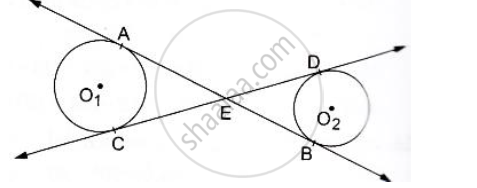Q 5

If PT is a tangent to a circle with center O and PQ is a chord of the circle such that ∠QPT = 70°, then find the measure of ∠POQ.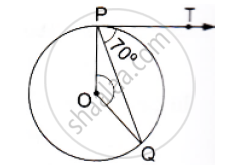Q 6

In the given figure, a triangle ABC is drawn to circumscribe a circle of radius 2 cm such that the segments BD and DC into which BC is divided by the point of contact D, are of lengths 4cm and 3cm respectively. If the area of 2 ABC  21cm then find the lengths of sides AB and AC.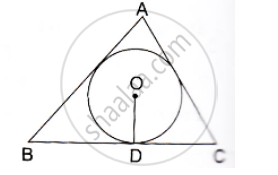Q 7

Two concentric circles are of radii 5cm and 3cm. Find the length of the chord of the larger circle (in cm) which touches the smaller circle.

Q 8

Prove that the perpendicular at the point of contact of the tangent to a circle passes through the centre.

Q 9

In the given figure, two tangents RQ, and RP and RP are drawn from an external point R to the circle with centre O. If ∠PRQ =120° , then prove that OR = PR + RQ.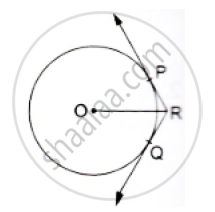Q 10

In the given figure, a cradle inscribed in a triangle ABC touches the sides AB, BC and CA at points D, E and F respectively. If AB = 14cm, BC = 8cm and CA=12 cm. Find the length AD, BE and CF.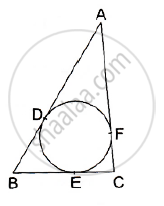Q 11

In the given figure, O is the centre of the circle. PA and PB are tangents. Show that AOBP  is cyclic quadrilateral.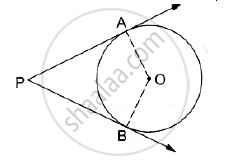Q 12

In two concentric circles, a chord of length 8cm of the large circle touches he smaller circle. If the radius of the larger circle is 5cm then find the radius of the smaller circle.

Q 13

In the given figure, PQ is chord of a circle with centre O an PT is a tangent. If
∠QPT = 60°, find the ∠PRQ.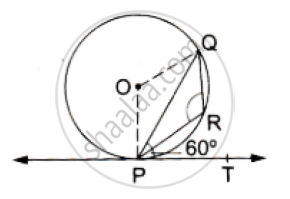Q 14

In the given figure, PA and PB are two tangents to the circle with centre O. If ∠APB = 60°  then find the measure of ∠OAB.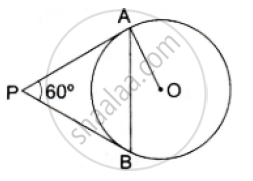## Chapter 12: Circles## RS Aggarwal solutions for Secondary School Class 10 Maths chapter 12 - Circles

RS Aggarwal solutions for Secondary School Class 10 Maths chapter 12 (Circles) include all questions with solution and detail explanation. This will clear students doubts about any question and improve application skills while preparing for board exams. The detailed, step-by-step solutions will help you understand the concepts better and clear your confusions, if any. Shaalaa.com has the CBSE Secondary School Class 10 Maths solutions in a manner that help students grasp basic concepts better and faster.

Further, we at Shaalaa.com provide such solutions so that students can prepare for written exams. RS Aggarwal textbook solutions can be a core help for self-study and acts as a perfect self-help guidance for students.

Concepts covered in Secondary School Class 10 Maths chapter 12 Circles are Circles Examples and Solutions, Introduction to Circles, Tangent to a Circle, Number of Tangents from a Point on a Circle, Circles Examples and Solutions, Introduction to Circles, Tangent to a Circle, Number of Tangents from a Point on a Circle.

Using RS Aggarwal Class 10 solutions Circles exercise by students are an easy way to prepare for the exams, as they involve solutions arranged chapter-wise also page wise. The questions involved in RS Aggarwal Solutions are important questions that can be asked in the final exam. Maximum students of CBSE Class 10 prefer RS Aggarwal Textbook Solutions to score more in exam.

Get the free view of chapter 12 Circles Class 10 extra questions for Secondary School Class 10 Maths and can use Shaalaa.com to keep it handy for your exam preparation